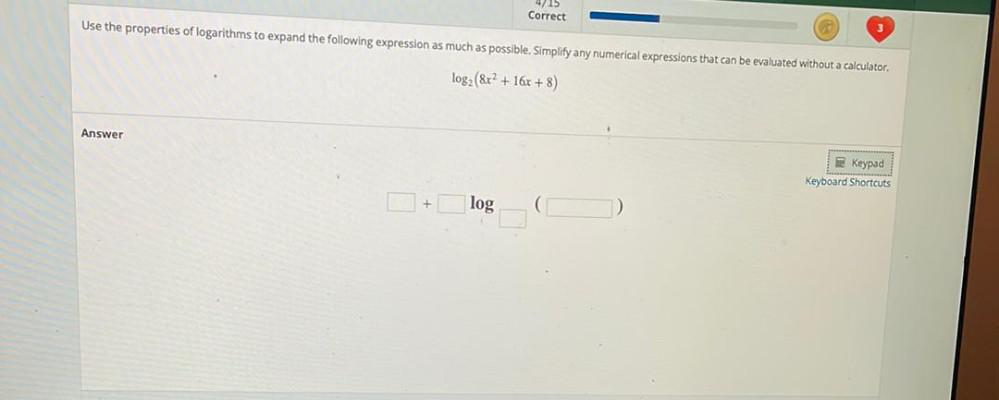Question:

# Correct - Use the properties of logarithms to expand the following expression as much as possible. Simplify any numerical expresCorrect - Use the properties of logarithms to expand the following expression as much as possible. Simplify any numerical expressions that can be evaluated without a calculator. log2 (&r? + 16x + 8) Answer Keypad Keyboard Shortcuts + log